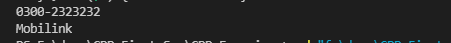Write a C++ program that should ask the user to input a phone number where the network and number are separated by a dash “-” like 0300-1234567. Then, print which network is used in that number. For simplicity, assume the following codes are used by the respective networks:

Code Network Name

0333 Ufone

0315 Zong

0345 Telenor

#include <iostream>
#include <string>

using namespace std;

void Network()
{
string network;
cin >> network;
for (int i = 0; i < network.length(); i++)
{
if (network.at(i) == '-')
{
network.erase(i, network.length());
}
if (network == "0300")
{
}
else if (network == "0333")
{
cout << "Ufone";
}
else if (network == "0315")
{
cout << "Zong";
}
else if (network == "0345")
{
cout << "Telenor";
}
}
}
int main()
{
Network();
return 0;
}cpp programming exercise with solution